Browse by Topic
Related Topics

# What Do Gravitational Crystals Really Look (i.e. Move) Like?

In a recent blog, Stephen Wolfram discusses the idea of what he calls “gravitational crystals.” These are infinite arrays of gravitational bodies in periodic motion. Two animations of mesmerizing movements of points were given as examples of what gravitational crystals could look like, but no explicit orbit calculations were given.

In this blog, I will carefully calculate explicit numerical examples of gravitational crystal movements. The “really” in the title should be interpreted as a high-precision, numerical solution to an idealized model problem. It should not be interpreted as “real world.” No retardation, special or general relativistic effects, stability against perturbation, tidal effects, or so on are taken into account in the following calculations. More precisely, we will consider the simplest case of a gravitational crystal: two gravitationally interacting, rigid, periodic 2D planar arrays embedded in 3D (meaning a 1/distance2 force law) of masses that can move translationally with respect to each other (no rotations between the two lattices). Each infinite array can be considered a crystal, so we are looking at what could be called the two-crystal problem (parallel to, and at the same time in distinction to, the classical gravitational two-body problem).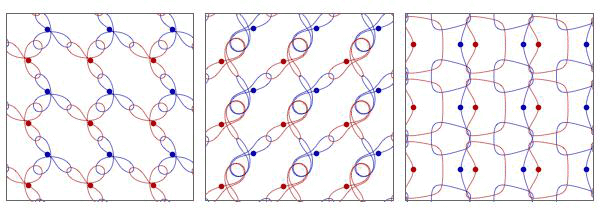Crystals have been considered for centuries as examples of eternal, never-changing objects. Interestingly, various other time-dependent versions of crystals have been suggested over the last few years. Shapere and Wilczek suggested space-time crystals in 2012, and Boyle, Khoo, and Smith suggested so-called choreographic crystals in 2014.

In the following, I will outline the detailed asymptotic calculation of the force inside a periodic array of point masses and the numerical methods to find periodic orbits in such a force field. Readers not interested in the calculation details should fast-forward to the interactive demonstration in the section “The resulting gravitational crystals.”

#### The force of a square grid of masses

Within an infinite crystal-like array of point masses, no net force is exerted on any of the point masses due to symmetry cancellation of the forces of the other point masses. This means we can consider the whole infinite array of point masses as rigid. But the space between the point masses has a nontrivial force field.

To calculate orbits of masses, we will have to solve Newton’s famous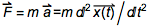. So, we need the force of an infinite array of 1/r potentials. We will consider the simplest possible case, namely a square lattice of point masses and lattice constant L. The force at a point {x,y} is given by the following double sum: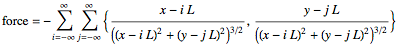Unfortunately, we can’t sum this expression in closed form. Using the sum of the potential is not easier, either; it actually increases the likelihood of a complication in the form of the potential diverging. (Although deriving and subtracting the leading divergent term is possible: if we truncate the sums at ±M, we have a linearly divergent term 8 M sinh-1(1).)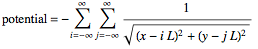So one could consider a finite 2D array of (2M+1)×(2M+1) point masses in the limit M→∞.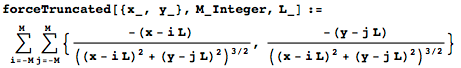But the convergence of the double sum is far too slow to get precise values for the force. (We want the orbit periodicity to be correct to, say, 7 digits. This means we need to solve the differential equation to about 9 digits, and for this we need the force to be correct to at least 12 digits.)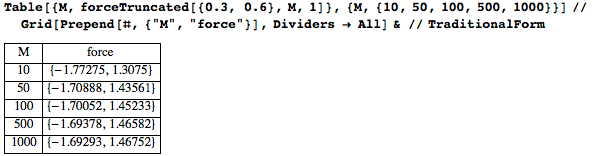Because the force is proportional to 1/distance2, and the number of point masses grows with distance squared, taking all points into account is critical for a precise force value. Any approximation can’t make use of a finite number of point masses, but must instead include all point masses.

Borrowing some ideas from York and Wang, Lindbo and Tornberg, and Bleibel for calculating the Madelung constant to high precision, we can make use of one of the most popular integrals in mathematics.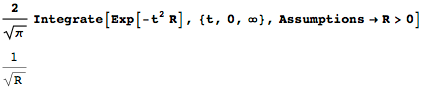This allows us to write the force as: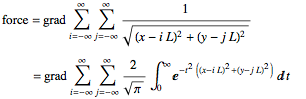Exchanging integration and summation, we can carry out the double sum over all (2∞+1)2 lattice points in terms of elliptic theta functions.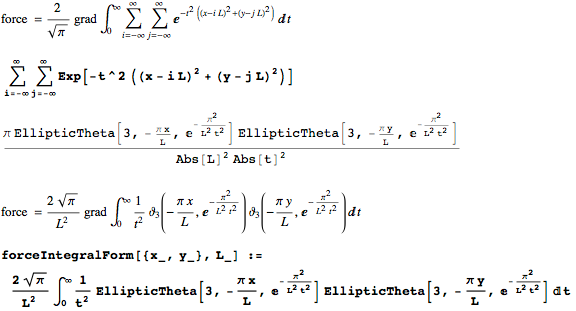Here we carry out the gradient operation under the integral sign: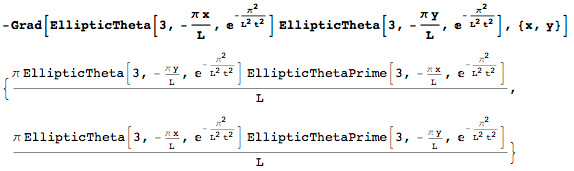We obtain the following converging integral: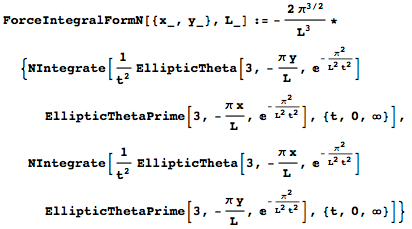While the integral does converge, numerical evaluation is still quite time consuming, and is not suited for a right-hand-side calculation in a differential equation.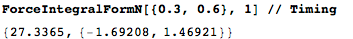Now let’s remind ourselves about some properties of the Jacobi elliptic theta function 3. The two properties of relevance to us are its sum representation and its inversion formula.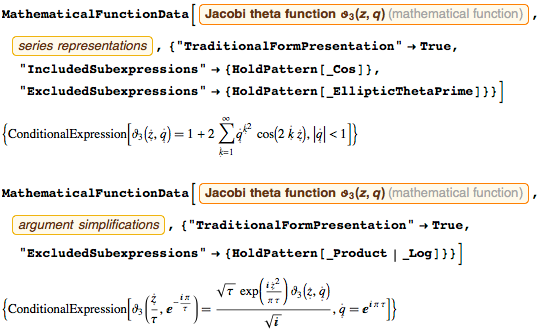The first identity shows that for t→0, the theta function (and its derivative) vanishes exponentially. The second identity shows that exponential decay can also be achieved at t→∞.

Using the sum representation, we can carry out the t integration in closed form after splitting the integration interval in two parts. As a result, we obtain for the force a sum representation that is exponentially convergent.

After some lengthy algebra, as one always says (which isn’t so bad when using the Wolfram Language, but is still too long for this short note), one obtains a formula for the force when using the above identities for ϑ3 and similar identities for ϑ´3. Here is the x component of the force. Note that M is now the limit of the sum representation of the elliptic theta function, not the size of the point mass lattice. The resulting expression for the force components is pretty large, with a leaf count of nearly 4,000. (Open the cell in the attached notebook to see the full expression.)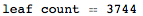Here is a condensed form for the force in the x direction that uses the abbreviation
ri j = (x + i L)2 + (y + j L)2: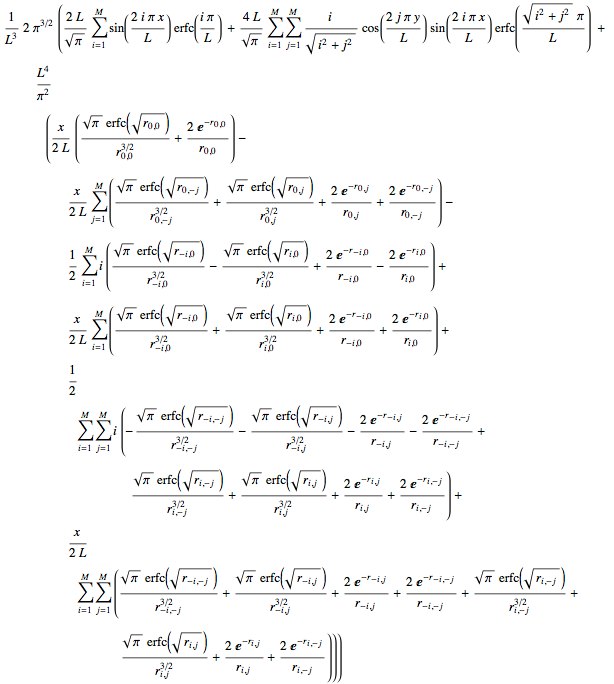Truncating the exponentially convergent sums shows that truncation at around 5 terms gives about 17 correct digits for the force.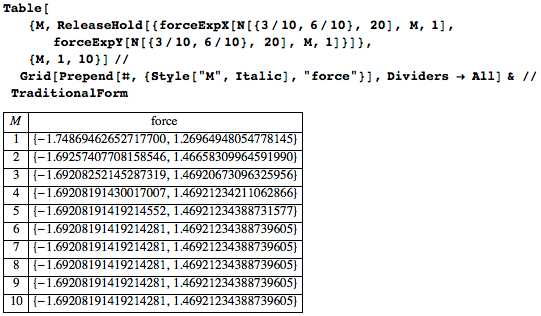The convergence speed is basically independent of the position {x, y}. In the next table, we use a point on the diagonal near to the point mass at the origin of the coordinate system.For points near to a point mass, we recover, of course, the 1/distance2 law.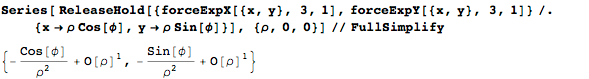For an even faster numerical calculation of the force, we drop higher-order terms in the double sums and compile the force.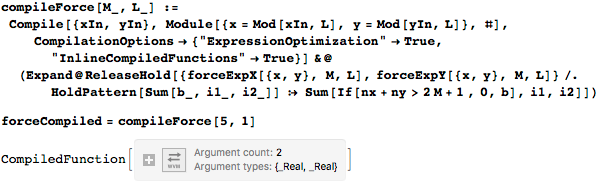All digits of the force are correct to machine precision.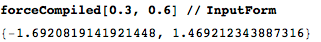And the calculation of a single force value takes about a tenth of a millisecond, which is well suited for further numerical calculations.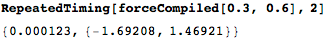For further use, we define the function forceXY that for approximate position values returns the 2D force vector.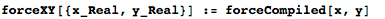#### The space of possible orbits

So now that we have a fast-converging series expansion for the force for the full infinite array of point masses, we are in good shape to calculate orbits.

The simplest possible situation is two square lattices of identical lattice spaces with the same orientation, moving relative to each other. In this situation, every point mass of lattice 1 experiences the same cumulative force from lattice 2, and vice versa. And within each lattice, the total force on each point mass vanishes because of symmetry.

Similar to the well-known central force situation, we can also separate the center of mass from the relative motion. The result is the equation of motion for a single mass point in the field of one lattice.

Here is a plot of the magnitude of the resulting force.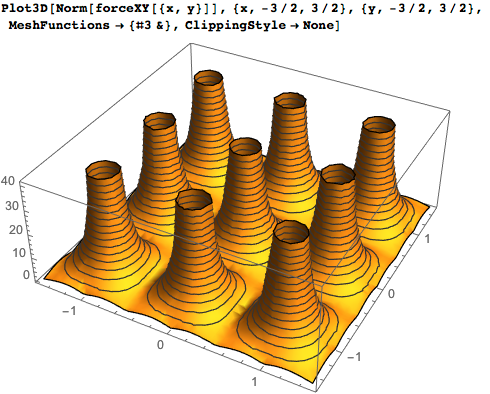And here is a plot of the direction field of the force. The dark red dots symbolize the positions of the point masses.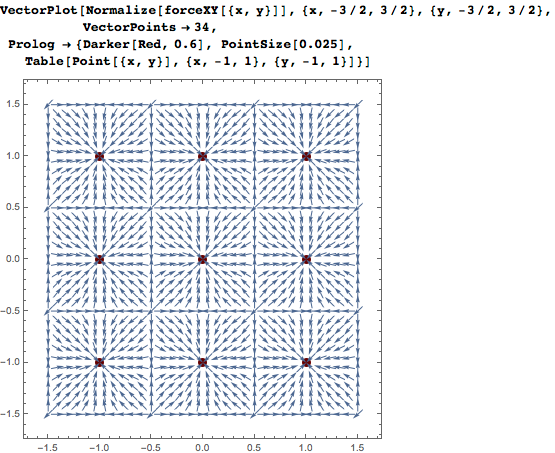How much does the field strength of the periodic array differ from the field strength of a single point mass? The following graphic shows the relative difference. On the horizontal and vertical lines in the middle of the rows and columns of the point masses, the difference is maximal. Due to the singularity of the force at the point masses, the force of a single mass point and the one of the lattice become identical in the vicinity of a point mass.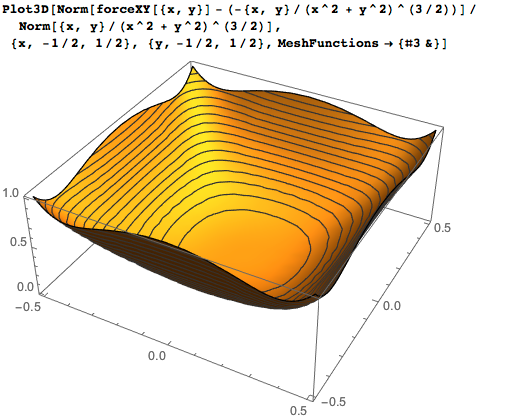The next plot shows the direction field of the difference between a single point mass and the periodized version.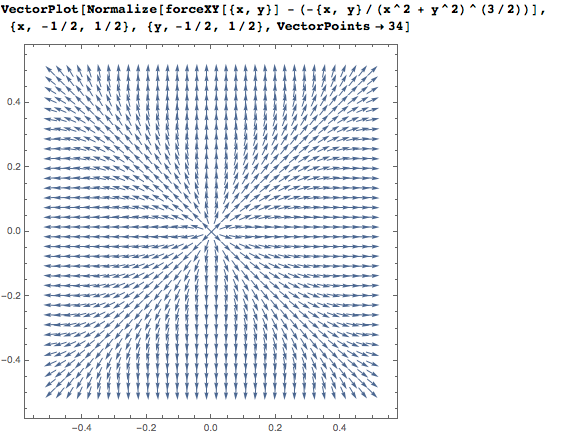Once we have the force field, inverting the relation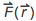=-grad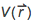numerically allows us (because the force is obviously conservative) to calculate the potential surface of the infinite square array of point masses.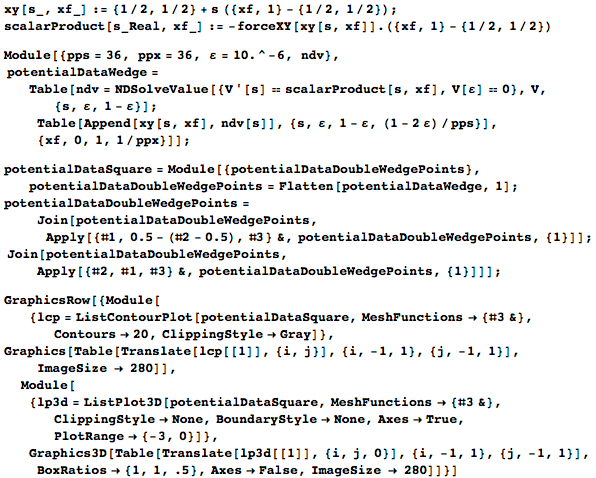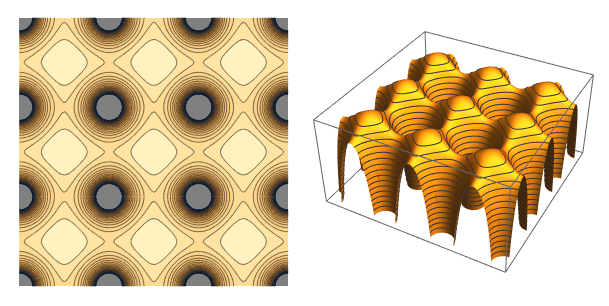Now lets us look at actual orbits in the potential shown in the last two images.

The following Manipulate allows us to interactively explore the motion of a particle in the gravitational field of the lattice of point masses.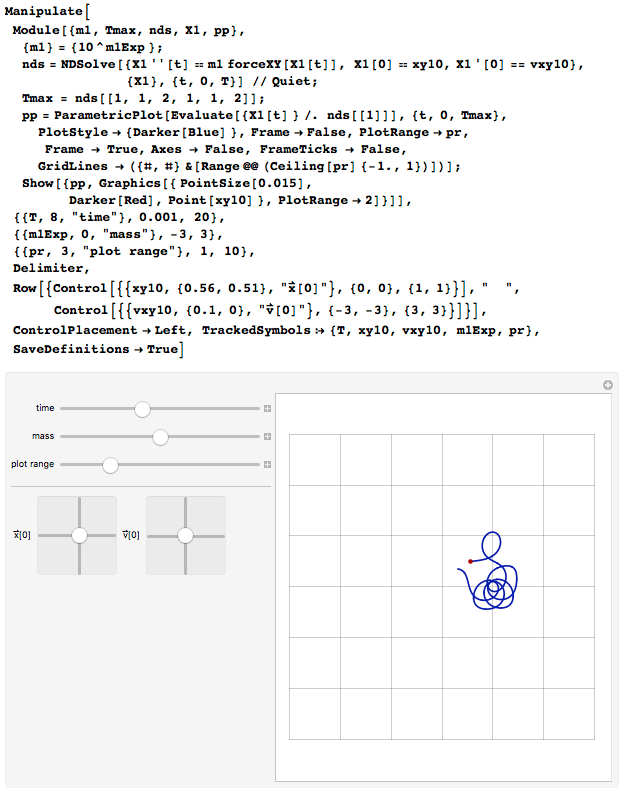The relatively large (five-dimensional) space of possible orbits becomes more manageable if we look especially for some symmetric orbits, e.g. we enforce that the orbit crosses the line x = 0 or
x = 1/2 horizontally. Many orbits that one would intuitively expect to exist that move around 1, 2, or 3 point masses fall into this category. We use a large 2D slider to allow a more fine-grained control of the initial conditions.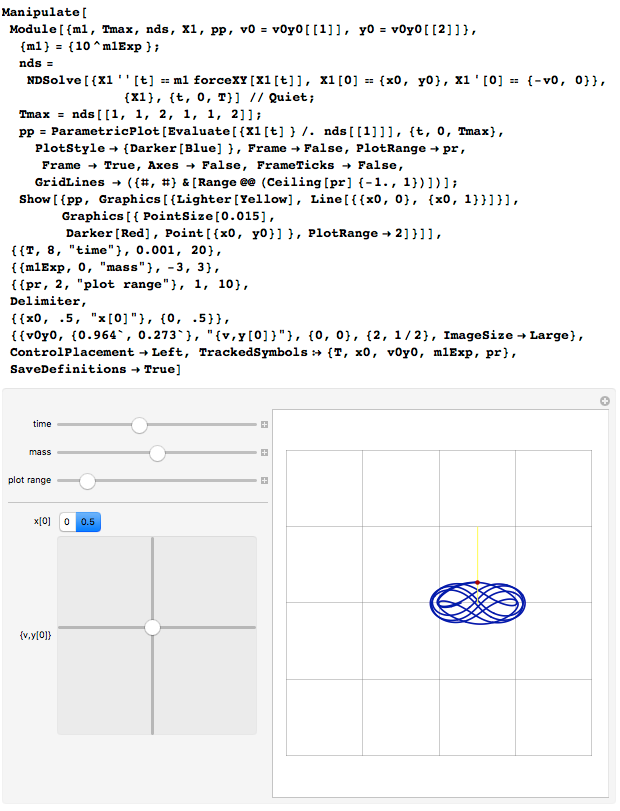Another highly symmetric situation is a starting point along the diagonal with an initial velocity perpendicular to it.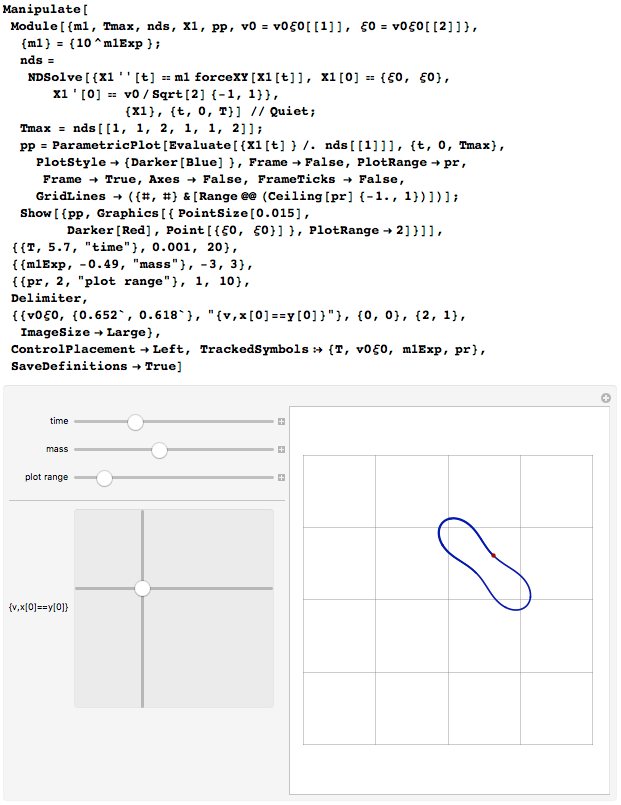#### Finding periodic orbits

For the desired motion we are looking for, we demand that after a period, the particle comes back to either its original position with its original velocity vector or has moved to an equivalent lattice position.

Given an initial position, velocity, mass, and approximate period, it is straightforward to write a simple root-finding routine to zoom into an actual periodic orbit. We implement this simply by solving the differential equation for a time greater than the approximate orbit time, and find the time where the sum of the difference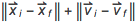of the initial and final positions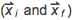and initial and final velocities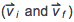is minimal. The function findPeriodicOrbit carries out the search. This method is well suited for orbits whose periods are not too long. This will yield a nice collection of orbits. For longer orbits, errors in the solution of the differential equation will accumulate, and more specialized methods could be employed, e.g. relaxation methods.

Given some starting values, findPeriodicOrbit attempts to find a periodic orbit, and returns the corresponding initial position and velocity.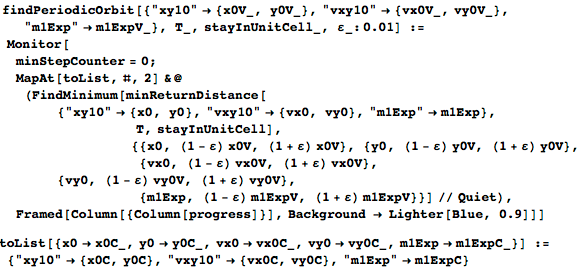Given initial conditions and a maximal solution time, the function minReturnData determines the exact time at which the differences between the initial and final positions and velocities are minimal. The most time-consuming step in the search process is the solution of the differential equation. To avoid repeating work, we do not include the period time as an explicit search variable, but rather solve the differential equation for a fixed time T and then carry out a one-dimensional minimization to find the time at which the sum of the position and velocity differences becomes minimal.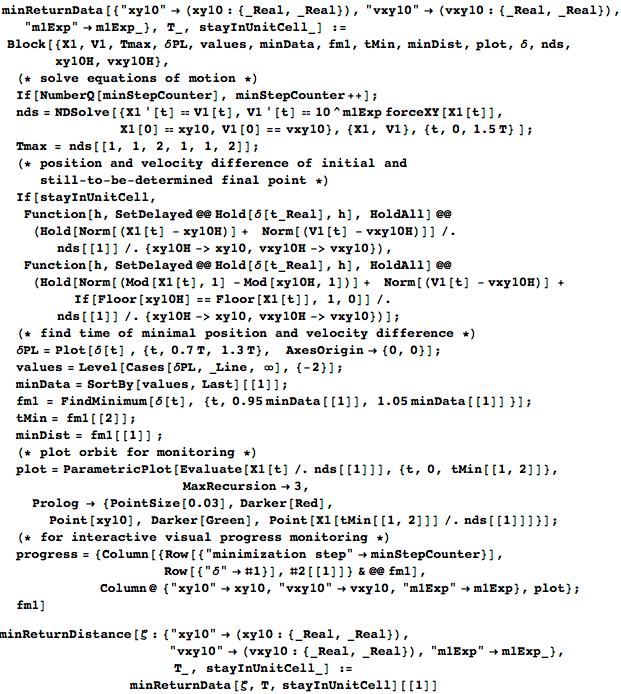As the search will take about a minute per orbit, we monitor the current orbit shape to entertain us while we wait. Typically, after a couple hundred steps we either find a periodic orbit, or we know that we failed to find a periodic orbit. In the latter case, the local minimum of the function to be minimized (the sum of the norms of initial versus final positions and velocities) has a finite value and so does not correspond to a periodic orbit.

Here is a successful search for a periodic orbit. The initial conditions for the search we either get interactively from the above Manipulate or from a random search selecting viable candidate initial conditions.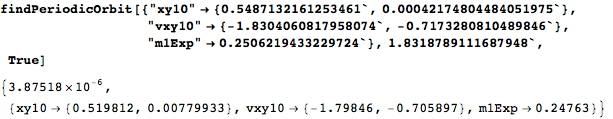Here is a successful search for an orbit that ends at an equivalent lattice position.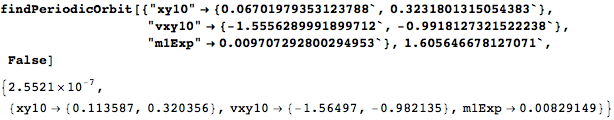So what kind of periodic orbits can we find? As the result of about half a million solutions with random initial positions, velocities, masses, and solution times of the equations of motion, we find the following types of solutions:

1. Closed orbits around a single point mass

2. Closed orbits around a finite (≥ 2) point mass

3. “Traveling orbits” that don’t return to the initial position but to an equivalent position in another lattice cell

(In this classification, we ignore “head-on” collision orbits and separatrix-like orbits along the symmetry lines between rows and columns of point masses.)

Here is a collection of initial values and periods for periodic orbits found in the carried-out searches. The small summary table gives the counts of the orbits found.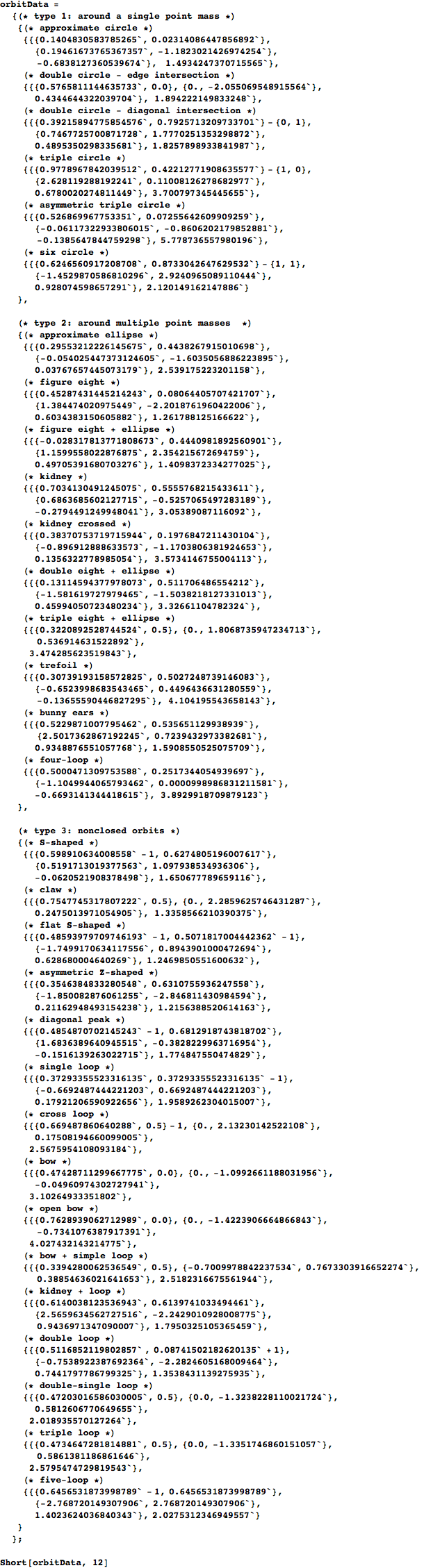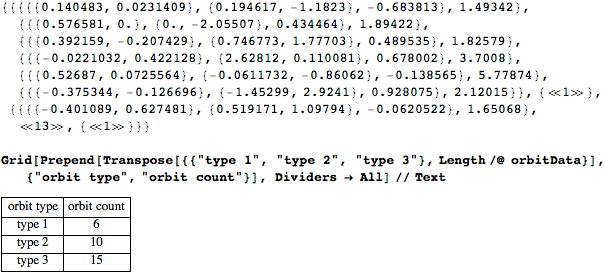Using minReturnDistance, we can numerically check the accuracy of the orbits. At the “return time” (the last element of the sublists of orbitData), the sum of the differences of the position and velocity vectors is quite small.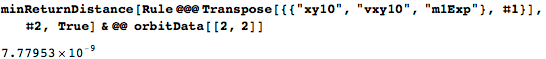Now let’s make some graphics showing the orbits from the list orbitData using the function showOrbit.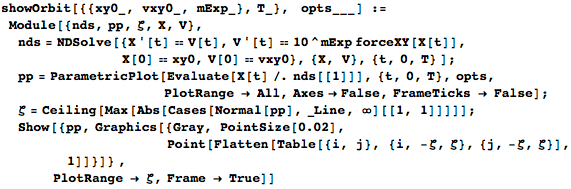1. Orbits around a single point mass

In the simplest case, these are just topologically equivalent to a circle. This type of solution is not unexpected; for initial conditions close to a point mass, the influence of the other lattice point masses will be small.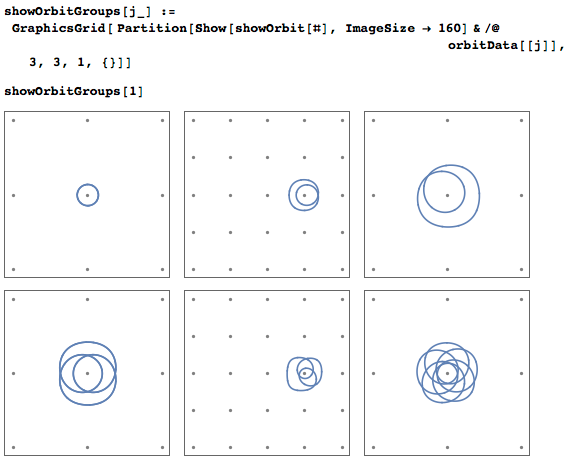2. Orbits around two point masses

In the simplest case, these are again topologically equivalent to a circle, but more complicated orbits exist. Here are some examples.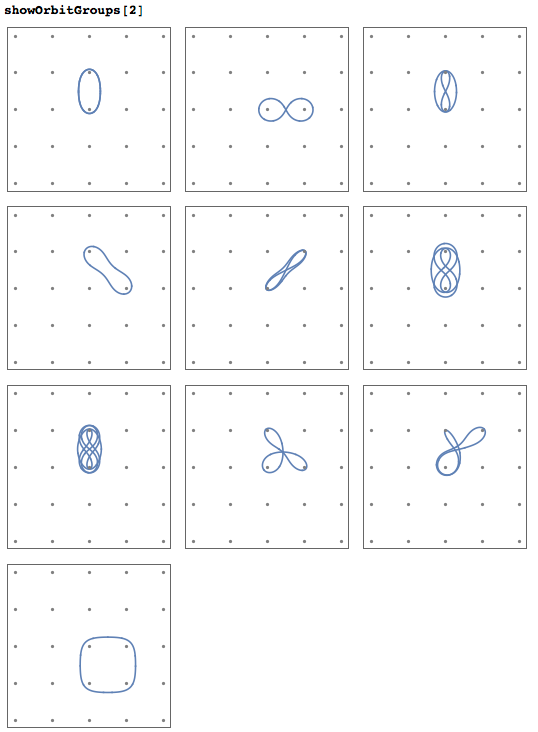3. “Traveling orbits” (open orbits) that don’t return to the initial position but to an equivalent position in another lattice cell

These orbits come in self-crossing and non-self-crossing versions. Here are some examples.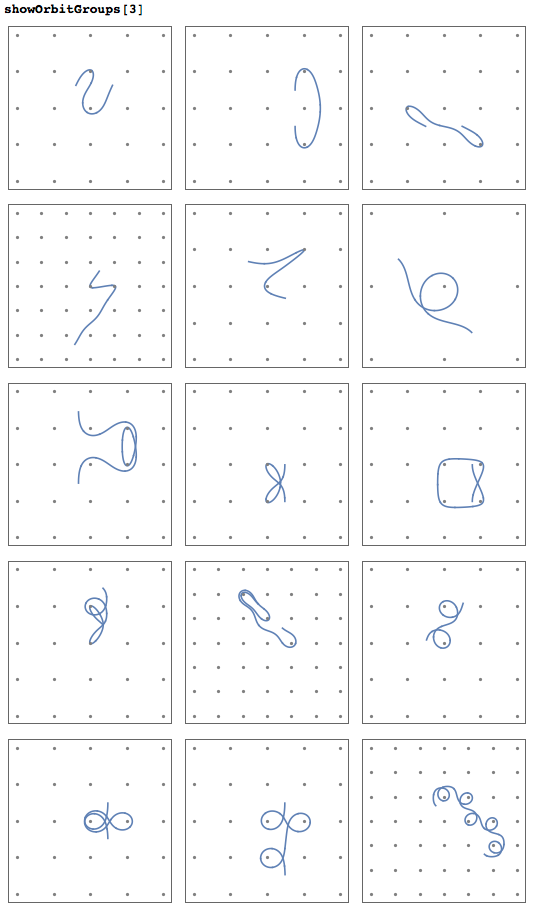Individually, the open orbits look quite different from the closed ones. When plotting the continuations of the open orbits, their relation to the closed orbits becomes much more obvious.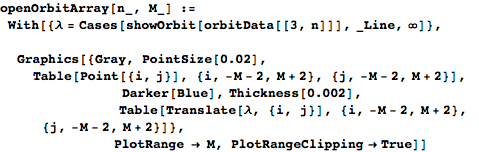For instance, the following open orbit reminds me of the last closed orbit.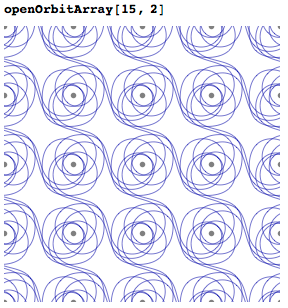The last graphic suggests that closed orbits around a finite number of points could become traveling orbits after small perturbations by “hopping” from a closed orbit around a single or finite number of point masses to the next single or finite group of point masses.

But there are also situations where one intuitively might expect closed orbits to exist, but numerically one does not succeed in finding a precise solution. One example is a simple rounded-corner, triangle-shaped orbit that encloses three point masses.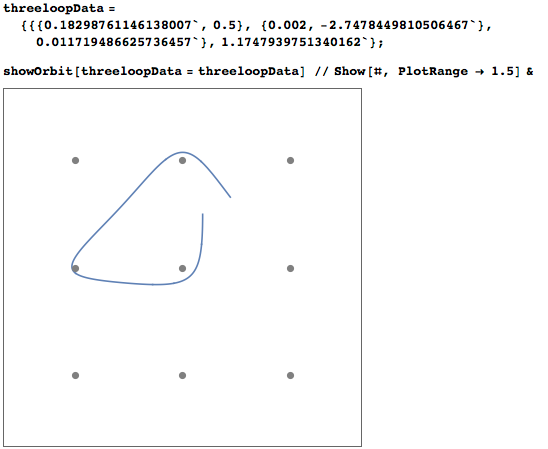Showing 100 orbits with slightly disturbed initial conditions gives an idea of why a smooth match of the initial point and the final point does not work out. While we can make the initial and final point match, the velocity vectors do not agree in this case.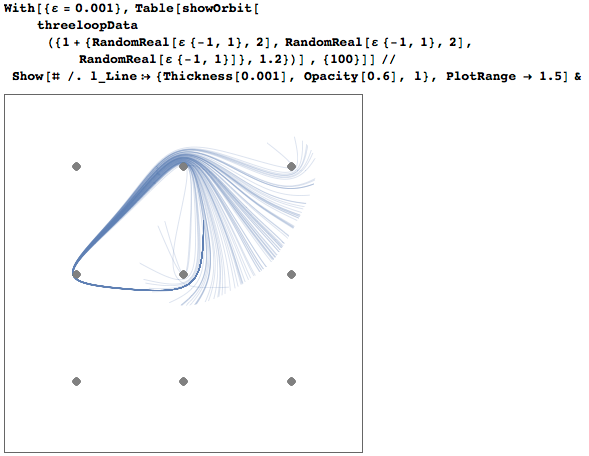Another orbit that seems not to exist, although one can make the initial and final points and velocities match pretty well, is the following double-slingshot orbit. But reducing the residue further by small modifications of the initial position and velocity seems not to be possible.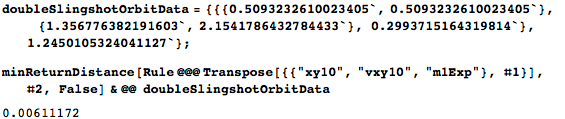Here are a third and fourth type of orbit that nearly match up, but the function findPeriodicOrbit can’t find parameters that bring the difference below 10-5.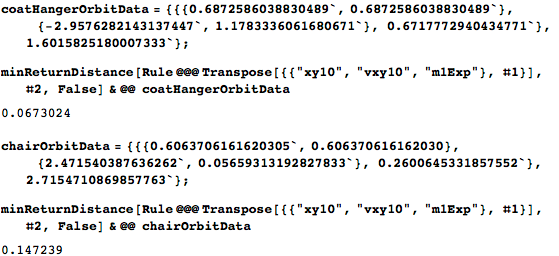Here are two graphics of the last two orbits.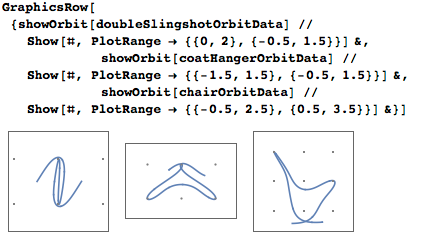There are many more periodic orbits. The above is just a small selection of all possible orbits. Exploring a family of trajectories at once shows the wide variety of orbits that can arise. We let all orbits start at the line segment {{x, 1/2}|-1/2 ≤ x ≤ 1/2} with an angle α(x) = 𝜋(1/2-|x|).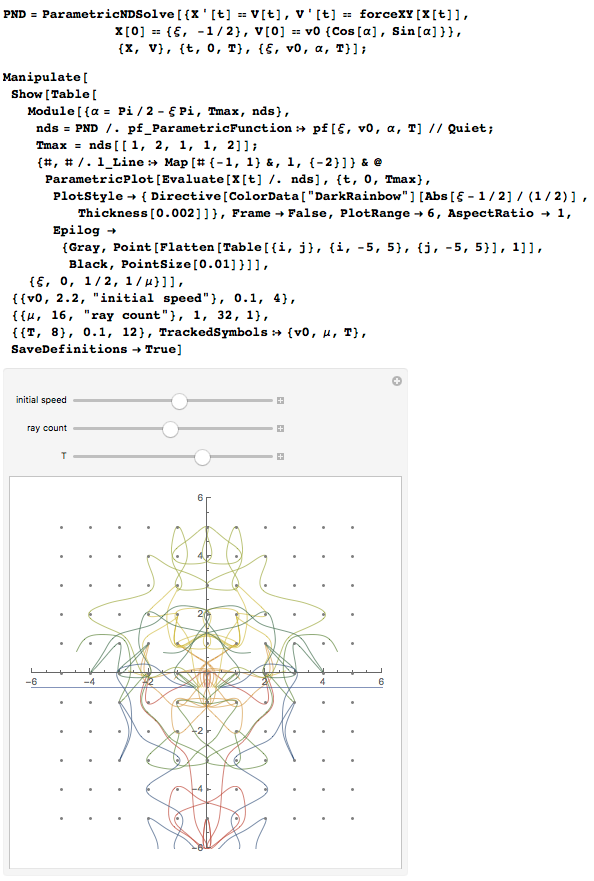If we plot sufficiently many orbits and select the ones that do not move approximately uniformly, we can construct an elegant gravitational crystal church.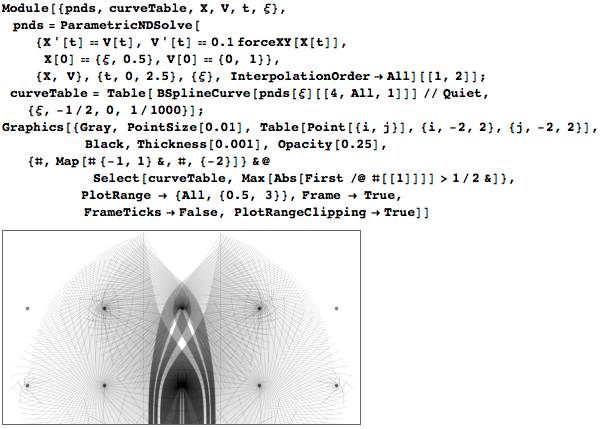The last image nicely shows the “branching” of the trajectories at point masses where the overall shape of the trajectory changes discontinuously. Displaying the flow in the three-dimensional xty space shows the branching even better.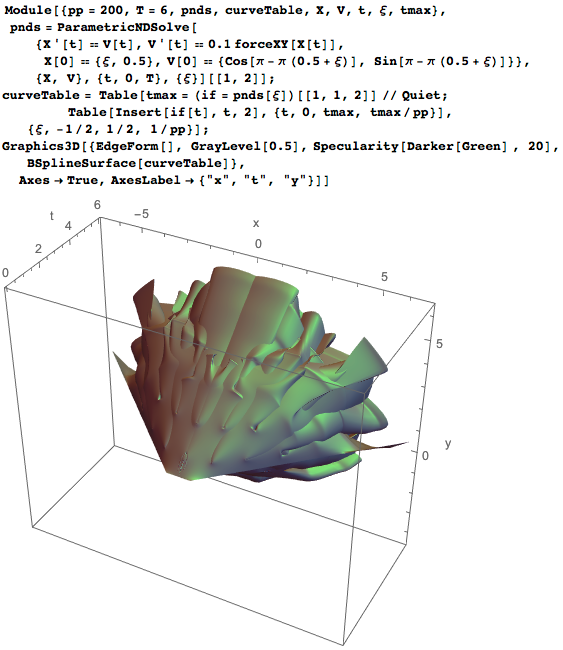#### General trajectories

We were looking for concrete periodic orbits in the field of an infinite square array of point masses. For more general results on trajectories in such a potential, see Knauf. Knauf proves that the behavior of average orbits is diffusive. Periodic orbits are the exception in the space of initial conditions. Almost all orbits will wander randomly around. So let’s have a quick look at a larger number of orbits. The following calculation will take about six hours, and evaluates the final points and velocities of masses starting at {x,0.5} with a velocity {0,v} on a dense xv grid with 0 ≤ x ≤ 1 and 1 ≤ v ≤ 3.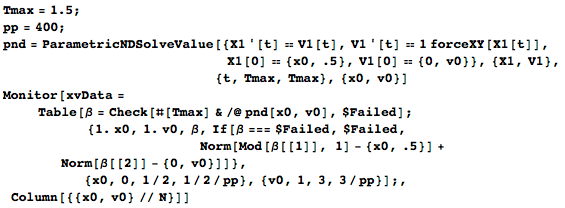If we display all final positions, we get the following graphic that gives a feeling of the theoretically predicted diffusive behavior of the orbits.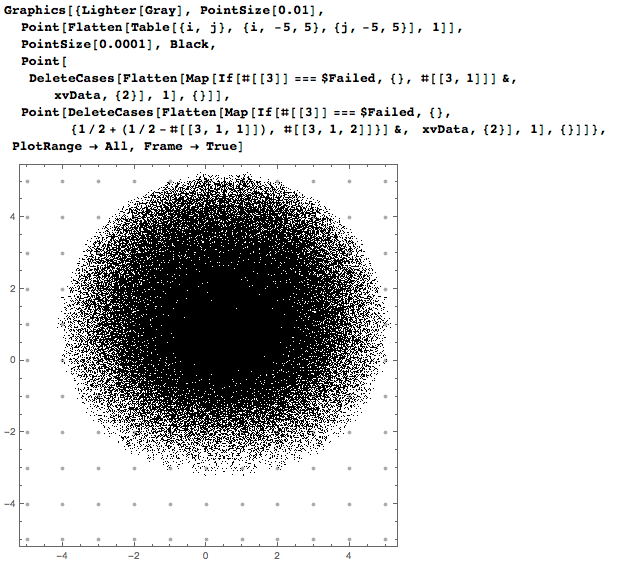While diffusive in average, as we are solving a differential equation, we expect (at least piecewise) that the final positions depend continuously on the initial conditions. So we burn another six hours of CPU time and calculate the final positions of 800,000 test particles that start radially from a circle around a lattice point mass. (Because of the symmetry of the force field, we have only 100,000 different initial value problems to solve numerically.)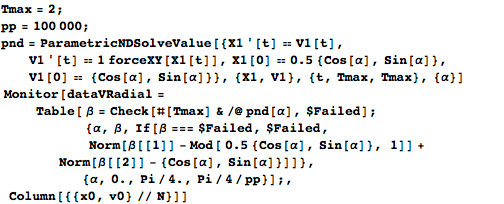Here are the points of the final positions of the 800,000 points. We again see how nicely the point masses of the lattice temporarily deflect the test masses.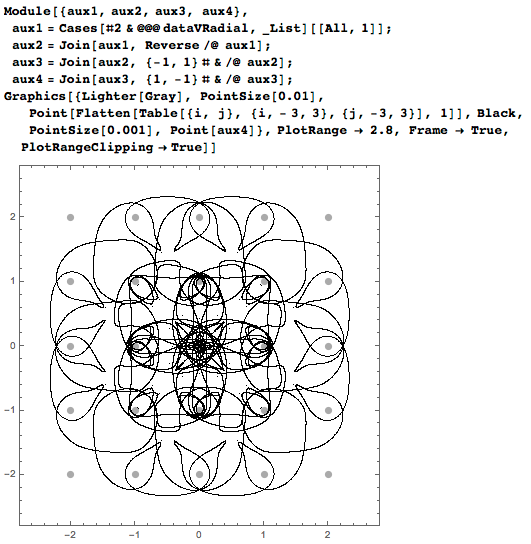We repeat a variation of the last calculation and determine the minimum value of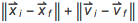in the xv plane, where x and v are the initial conditions of the particle starting at y = 0.5 perpendicular upward.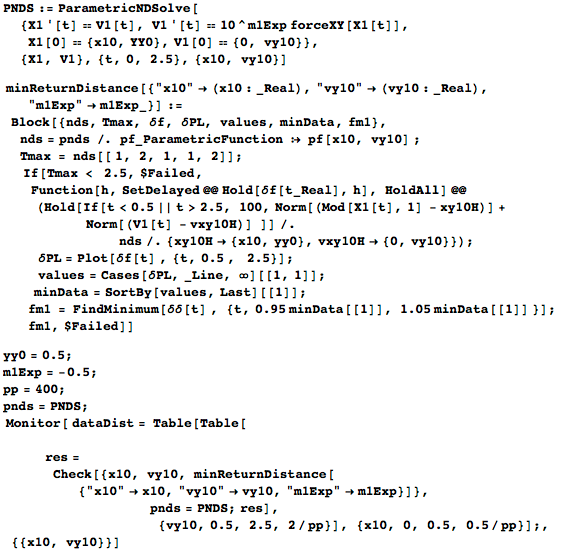We solve the equations of motions for 0 ≤ t ≤ 2.5 and display the value of the minimum ofin the time range 0.5 ≤ t ≤ 2.5. If the minimum occurs for t=0.5, we use a light gray color; if the minimum occurs for t=2.5, a dark gray color; and for 0.5 < t < 2.5, we color the sum of norms from pink to green. Not unexpectedly, the distance sum shows a fractal-like behavior, meaning the periodic orbits form a thinly spaced subset of initial conditions.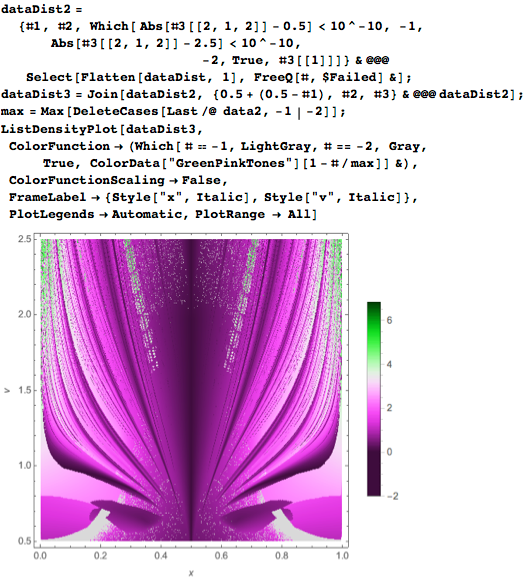#### A (2D) grain of salt

Now that we have the force field of a square array of point masses, we can also use this force to model electrostatic problems, as these obey the same force law.

Identical charges would form a Wigner crystal, which is hexagonal. Two interlaced square lattices of opposite charges would make a model for a 2D NaCl salt crystal.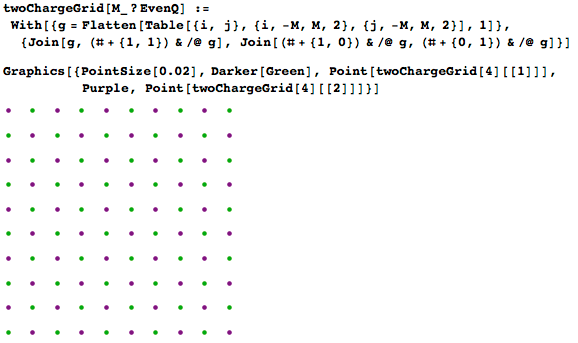By summing the (signed) forces of the four sublattices, we can again calculate a resulting force of a test particle.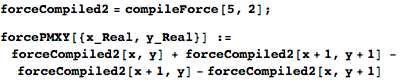The trajectories of a test charge become more irregular as compared with the gravitational model considered above. The following Manipulate allows us to get a feeling for the resulting trajectories. The (purple) test charge is attracted to the green charges of the lattice and repelled from the purple charges of the lattice.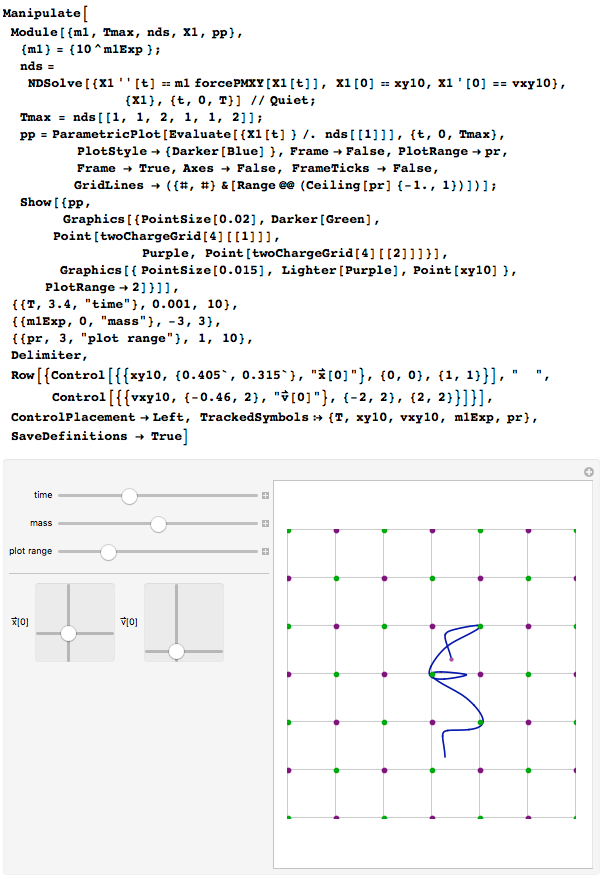#### The resulting gravitational crystals

We can now combine all the elements together to visualize the resulting gravitational crystals. We plot the resulting lattice movements in the reference frame of one lattice (the blue lattice). The red lattice moves with respect to the blue lattice.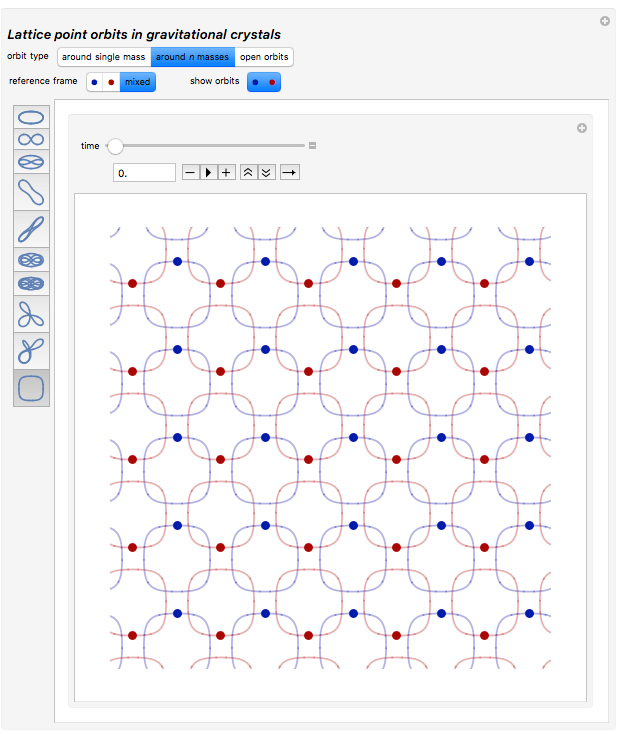#### Summary

Using detailed numerical calculation, we verified the existence of the suggested gravitational crystals. For the simplest case, the two square lattice, many periodic orbits of small period were found. More extensive searches would surely return more, longer period solutions.

Using the general form of the Poisson summation formula for general lattices, the above calculations could be extended to different lattices, e.g. hexagonal lattices or 3D lattices.